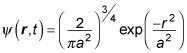##### Quantum Physics For Dummies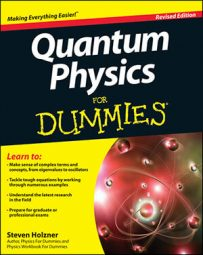At some point, your quantum physics instructor may want you to add time dependence and get a physical equation for a three-dimensional free particle problem. You can add time dependence to the solution for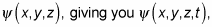if you remember that, for a free particle,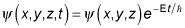That equation gives you this form for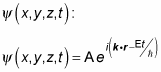Because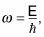the equation turns into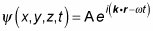In fact, now that the right side of the equation is in terms of the radius vector r, you can make the left side match: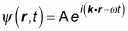That’s the solution to the Schrödinger equation, but it’s unphysical. Why? Trying to normalize this equation in three dimensions, for example, gives you the following, where A is a constant: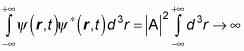(Remember that the asterisk symbol [*] means the complex conjugate. A complex conjugate flips the sign connecting the real and imaginary parts of a complex number. The limits on the integral just mean to integrate over all of space, like this: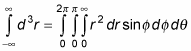Thus, the integral diverges and you can’t normalize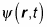as written here. So what do you do here to get a physical particle?

The key to solving this problem is realizing that if you have a number of solutions to the Schrödinger equation, then any linear combination of those solutions is also a solution. In other words, you add various wave functions together so that you get a wave packet, which is a collection of wave functions of the form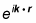such that

• The wave functions interfere constructively at one location.

• They interfere destructively (go to zero) at all other locations.

Look at the time-independent version: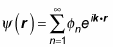However, for a free particle, the energy states are not separated into distinct bands; the possible energies are continuous, so people write this summation as an integral: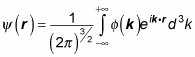So what is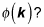It’s the three-dimensional analog of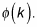That is, it’s the amplitude of each component wave function. You can find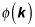from the Fourier transform of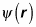like this: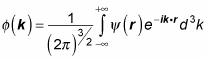In practice, you choose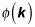yourself. Look at an example, using the following form forwhich is for a Gaussian wave packet (Note: The exponential part is what makes this a Gaussian wave form):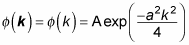where a and A are constants. You can begin by normalizingto determine what A is. Here’s how that works: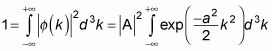Okay. Performing the integral gives you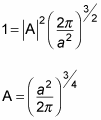which means that the wave function is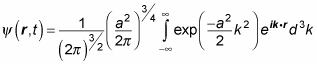You can evaluate this equation to give you the following, which is what the time-independent wave function for a Gaussian wave packet looks like in 3D: